News/Events

WAVEFORM MODULATION WITH YOUR FUNCTION GENERATOR

Basic function generators generally can generate basic periodic waveforms such as sine wave, square wave, triangle wave, ramp wave, pulse wave and so on. Modern function generators nowadays have a very important feature called modulation.

Advanced modulation features in modern function generators

Here, let us explore some advanced modulation features in modern function generators.

AM (Amplitude Modulation)

Amplitude modulation by a carrier sine wave is by far the most common regarding usage. Figure 1 shows the graphical settings of a function generator modulated by a sine wave with AM Depth of 100% and AM Frequency of 100 Hz. The original data sine wave has a frequency of 1 kHz.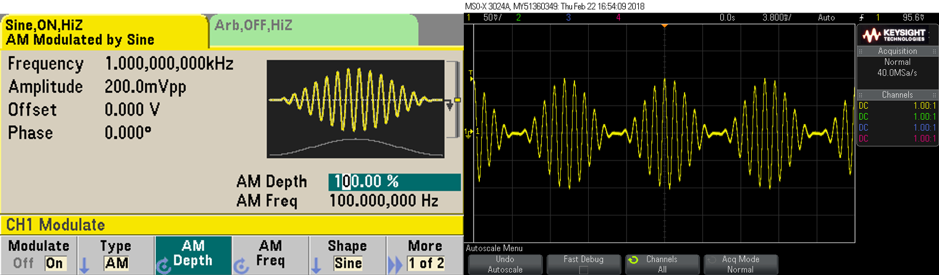Figure 1. AM function generator setup (left) using a Keysight 33600A and an oscilloscope display of AM (right)

Modern function generators can do more than just generate sine wave as a carrier signal for waveform modulation. Figure 2 shows some examples of other types of signals that are used as carrier signals for waveform modulations (other than a sine wave).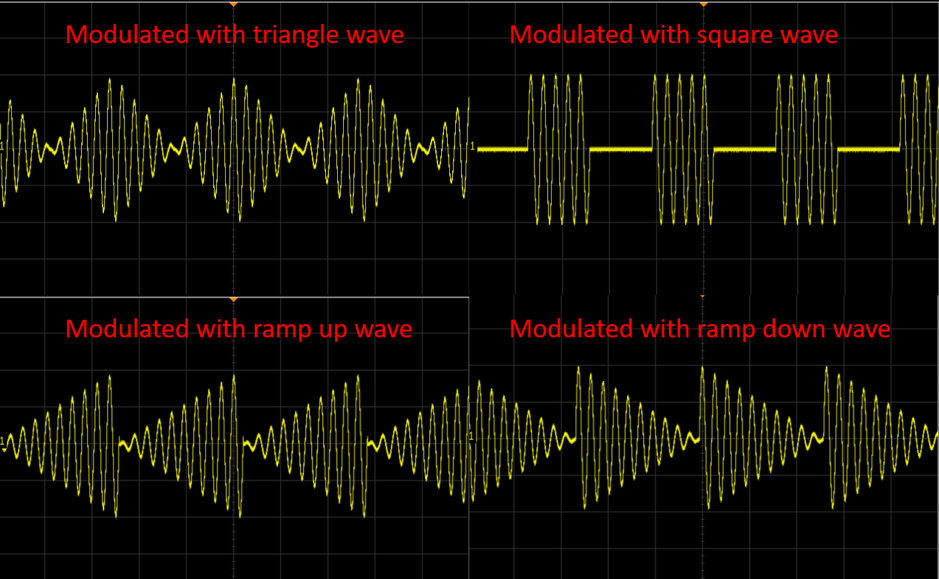Figure 2. Various carrier signals used for waveform modulations (other than a sine wave).

Modern function generators can be quite versatile. You can also choose the source of your AM modulation from another channel of your function generator without any external connections or from an external source. For example, you can use waveform from Channel 2 to modulate your waveform from Channel 1.

FM (Frequency Modulation)

Frequency modulation has been the best known analog modulation method for radio broadcasting for more than half a century. Its applications have grown into video broadcasting, critical medical monitoring systems, radar and more.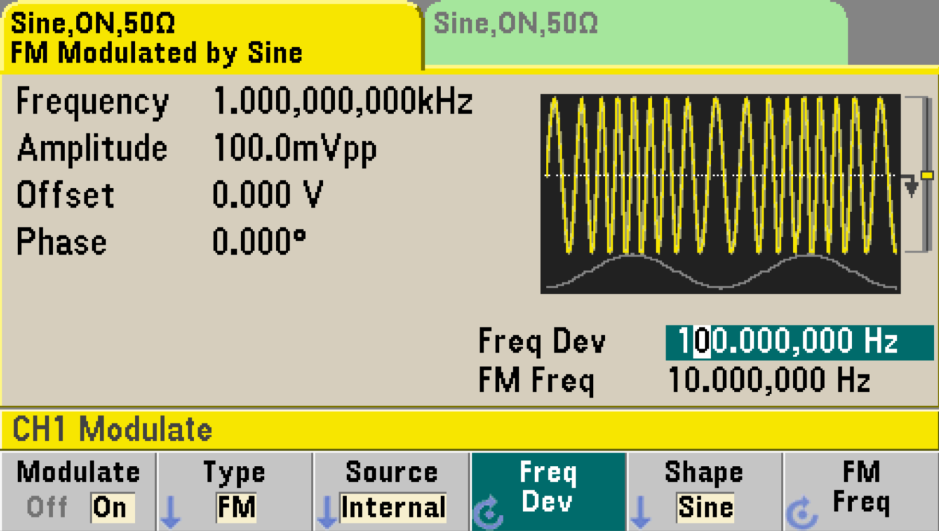Figure 3. FM function generator setup using a Keysight 33600A.

Here is an example of how to set up a function generator to simulate an FM signal. Figure 3 above shows frequency modulation on a 1 kHz sine wave. The modulation method used is also a sine wave with an FM frequency of 10 Hz. Its peak frequency deviation is 100 Hz.

PM (Phase Modulation)

Phase modulation is widely used in digital data transmissions through digital modulation techniques such as PSK (Phase-shift keying), BPSK (Binary phase-shift keying), QPSK (Quadrature phase-shift keying) and more. It is used in Wi-Fi, GSM, and satellite broadcasting transmissions.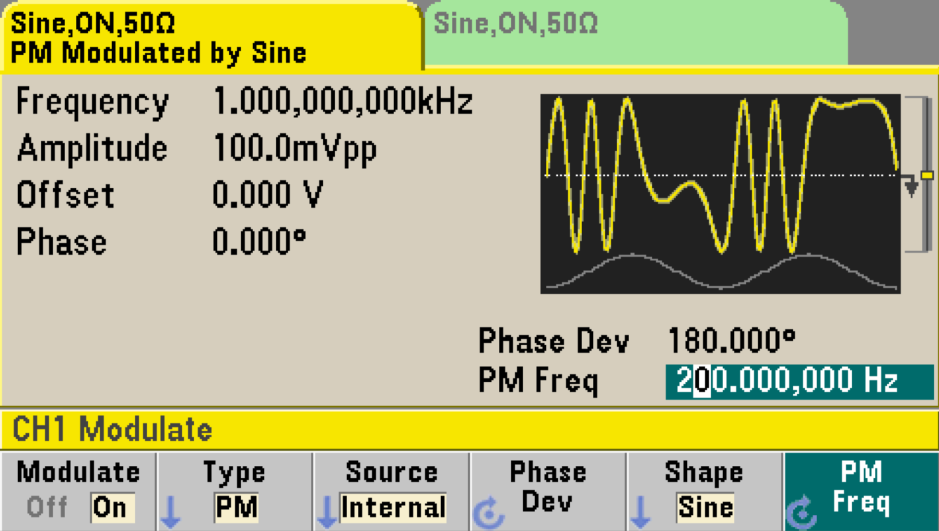Figure 4. PM function generator setup using a Keysight 33600A

Here is an example of how to set up a function generator to simulate a PM signal. Figure 4 above shows phase modulation on a 1 kHz sine wave. The modulation signal used is also a sine wave with a PM frequency of 200 Hz. Its phase deviation is 180°.

FSK (Frequency-shift keying) / BPSK (Binary phase-shift keying)

There are many types of digital modulation techniques available today. The common ones are FSK (frequency-shift keying) and BPSK (binary phase-shift keying). These digital modulation techniques are used in many applications such as digital radio transmissions, RFID, Bluetooth and more.Figure 5. FSK function generator setup using a Keysight 33600A

Here is an example of how to set up a function generator to simulate an FSK signal. Figure 5 above shows frequency-shift keying modulation on a 1 kHz carrier sine wave. The hop (or alternate) frequency is set at 100 Hz. The FSK rate is set at 10 Hz which is the rate at which output frequency "shifts" between the carrier and hop frequency.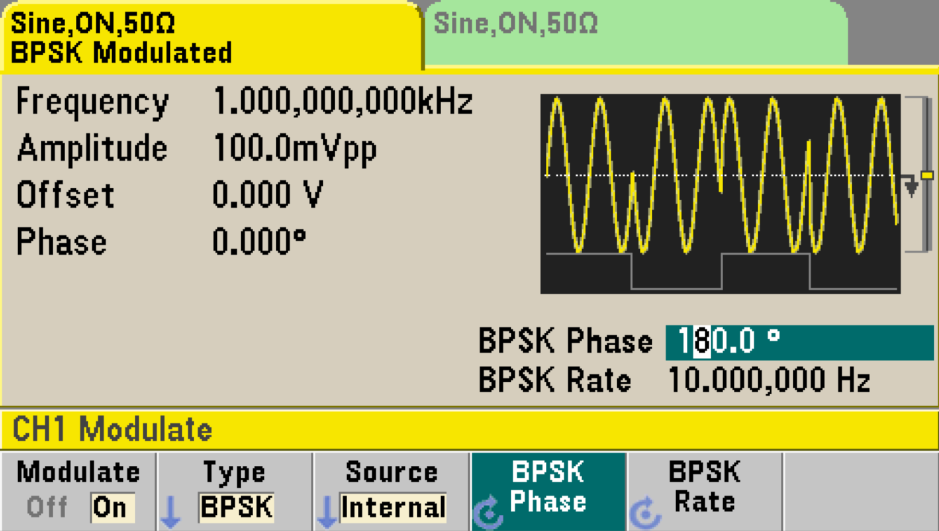Figure 6. BPSK function generator setup using a Keysight 33600A

Here is an example of how to set up a function generator to simulate a BPSK signal. Figure 6 above shows binary phase-shift keying modulation on a 1 kHz carrier sine wave. The BPSK rate is the rate at which the output phase "shifts" between the carrier and offset phase. The BPSK phase setting, in this case, is 180° (phase shift in degrees). Typical you have the option to set the phase shift from 0° to 360°.

SUM (Sum modulation)

Sum modulation adds a modulating signal to any carrier waveform; it is typically used to add Gaussian noise to a carrier. The modulating signal is added to the carrier as a percentage of carrier waveform amplitude.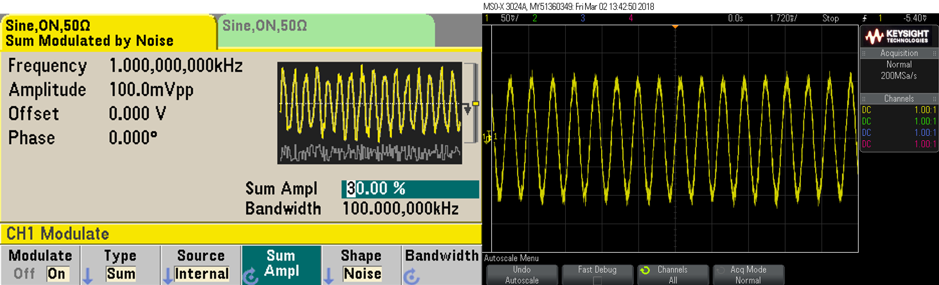Figure 7. Sum modulation function generator setup – adding noise  into a sine wave carrier signal.

The figure above shows how we can use the sum modulation to add measured noise to the carrier signal. This figure shows noise with an amplitude of 30% (referencing to carrier amplitude) and a noise bandwidth of 100 kHz was added to the sine wave carrier signal.

The Keysight 33600A series function generator accepts both internal and external modulation sources.  On a two-channel instrument, you can modulate one channel with the other. When using the internal source as modulation, we can select sine, square, triangle, up-ramp, down-ramp, noise, PRBS or arbitrary waveforms as the source of modulation.

Summary

We have just explored some advance modulation features and how intuitive the configuration setup can be using a Keysight 33600A.